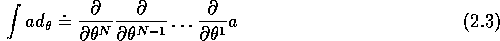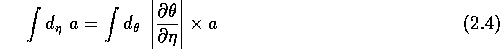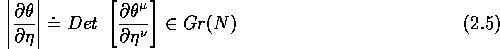Next: Derivations and differential forms Up: Differential forms over Grassmann Previous: Classical analogies

## Grassmann algebras (a compendium of basic results and notations)

We summarize here some properties of Grassmann algebras and introduce a few notations that we shall need in the sequel. More properties can be found in  or .

A Grassmann algebra Gr(N) can be defined as a (real or complex) associative unital algebra with N generators satisfying the defining relations and therefore In the following we choose the basic field as the field of complex numbers. As a vector space, it has dimension and a possible basis is where I is a multi-index with together with the convention when I=0. is a graded vector space but also a graded commutative algebra: Calling the intrinsic grade of a (i.e. 0 or 1 on even or odd product of resp.), we have It is sometimes useful to decompose Gr(N) as where denotes the space of nilpotents elements of Gr(N) and to notice that is an ideal in Gr(N). There is a canonical homomorphism from Gr(N) to , i.e., a character, defined by and This homomorphism is actually a unique nontrivial character in Gr(N). An element is invertible iff . In this case, calling one gets .

The generating system used to define Gr(N) is by no means unique; it is clear that one can make a change of generating system by using an invertible matrix with scalar coefficients but we should stress that one can also obtain new generating systems for the algebra Gr(N) by choosing coefficients in Gr(N) itself rather than in . More precisely, if one calls a chart (or frame) on Gr(N) a generating system such that the are odd and such that , one proves that these two conditions imply Let then be a frame of Gr(N), the corresponding Berezin integral is the element of the dual given bywhere In other words this Berezin integral is equal to , the coefficient of a at the ``top element'' . We have to stress the fact that the left hand side of the previous equation is defined by the right hand side; in particular the symbol has nothing to do with a 1-form : see section 2.3. If is another chart of Gr(N) -that we obtain from by an invertible matrix with coefficients in , the corresponding Berezin integral is defined in the same way but we have the relationwhereThe fact that both Berezin integrals associated with charts and are related in this way illustrates another interesting property of Grassmann algebras, namely the fact that the dual of Gr(N) is singly generated as a right module over Gr(N), in other words, if is such that then generates , i.e.Next: Derivations and differential forms Up: Differential forms over Grassmann Previous: Classical analogies

Robert Coquereaux
Mon May 20 14:40:14 MET DST 1996